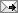## Sunday, November 9, 2008

### C0, C1, and C2 functionsFrom Wikipedia's Smooth Functions:

"The class C0 consists of all continuous functions. The class C1 consists of all differentiable functions whose derivative is continuous; such functions are called continuously differentiable."

A differentiable function might not be C1. The function f(x) = x^2*sin(1/x) for x \neq 0 and f(x) =0 for x=0 is everywhere continuous and differentiablem, but its derivative is f'(x) = -cos(1/x) + 2x*sin(1/x) for x \neq 0 and f'(x) =0 for x=0, which is discontinuous at x=0, so it is not C1.

Labels:

To view the post on a separate page, click: at (the permalink).